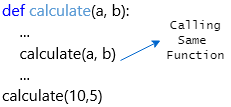# Python Recursive Function

Generally, recursion means calling the same thing itself. In python, the defined function can call itself within the same function definition, and we will call it a recursive function.

Following is the pictorial representation of calling the same function (recursive function) itself in python.While creating the recursive functions, you need to make sure that there is a condition that stops the recursion; otherwise, the function calls itself infinitely. You will get an exception like maximum recursion depth exceeded.

## Recursive Function Example

Following is the example of defining the recursive function in python.

def calculate(a, b):
if a > 0 and a <= 10:
x = a * b
print("{} * {} = {}".format(a, b, x))
calculate(a + 1, b)
calculate(1, 5)

If you observe the above example, we created the calculate function and we are calling the same calculate function inside of the function body.

When you execute the above python example, you will get the result as shown below.

1 * 5 = 5
2 * 5 = 10
3 * 5 = 15
4 * 5 = 20
5 * 5 = 25
6 * 5 = 30
7 * 5 = 35
8 * 5 = 40
9 * 5 = 45
10 * 5 = 50

As discussed, if you create a recursive function without any condition to stop the recursion you will get a recursion exception.

def calculate(a, b):
x = a * b
calculate(a, b)
print("Result:{}".format(x))
calculate(10,5)

When you execute the above python example, you will get an exception like as shown below.

Traceback (most recent call last):
File "", line 5, in
File "", line 3, in calculate
File "", line 3, in calculate
File "", line 3, in calculate
[Previous line repeated 1022 more times]
RecursionError: maximum recursion depth exceeded

This is how you can implement the function recursion in python to call the same function based on your requirements.